﻿ 求解区间非线性方程的一类改进算法 A Class of Improved Algorithm for Solving Interval Nonlinear Equation

Vol.06 No.05(2017), Article ID:21815,10 pages
10.12677/AAM.2017.65086

A Class of Improved Algorithm for Solving Interval Nonlinear Equation

Liang Qiu, Haijun Wang*, Qi Wang

School of Mathematics, China University of Mining and Technology, Xuzhou Jiangsu

*通讯作者。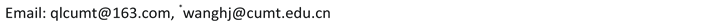Received: Aug. 6th, 2017; accepted: Aug. 20th, 2017; published: Aug. 25th, 2017ABSTRACT

In this paper, we consider the problem of solving interval nonlinear equation with interval parameters. Dividing the initial interval by monotone segment technique, we extended the improved interval Newton algorithm proposed in  , and established a class of improved algorithm for solving interval nonlinear equation. Besides, some relevant theoretical results and effectiveness tests are given. Numerical examples show the new algorithm can not only solve problems that can not be solved by improved interval Newton method, but also greatly improve the computational efficiency.

Keywords:Interval Nonlinear Equation with Interval Parameters, Improved Interval Newton Algorithm, Subinterval, Interval Zero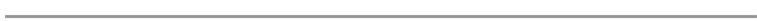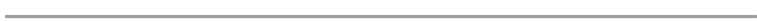1. 引言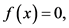(1)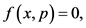(2)(3)

1966年，Moore  在《Interval Analysis》一书中第一次用区间牛顿法逼近了一个简单的区间方程的零解；1992年，Hansen  利用牛顿法提出了区间搜索和启发式的终止条件求解区间方程的零解；2006年，Nikas  等，在理论上提出了一种边界近似的方法求解区间方程，并取得了很好的成果；2009年，Nikas  等，又基于牛顿法提出了通过分离零解区间端点的方法求解区间方程。

2. 拓展的区间牛顿法(EIN)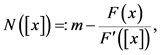(4)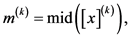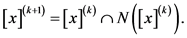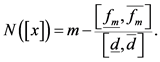(5)(6)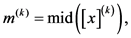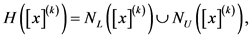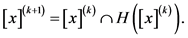(7)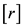总是存在且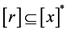(详见图1)。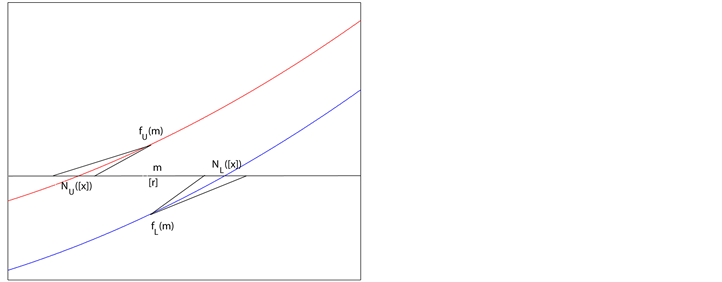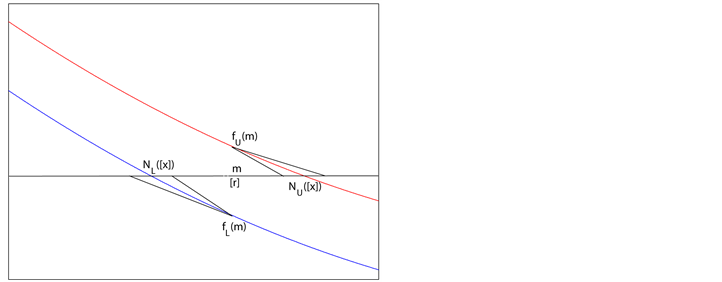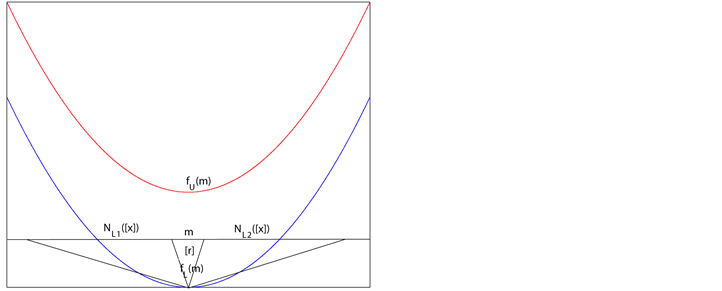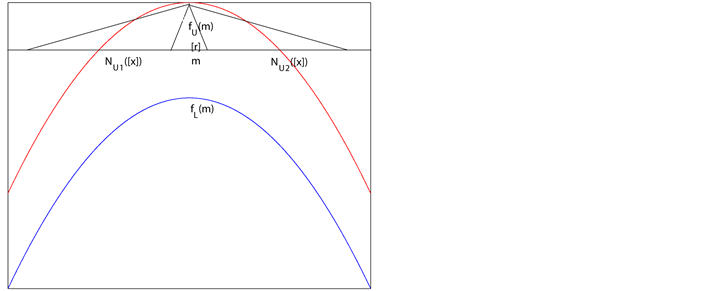Figure 1. Four cases in lemma 2.1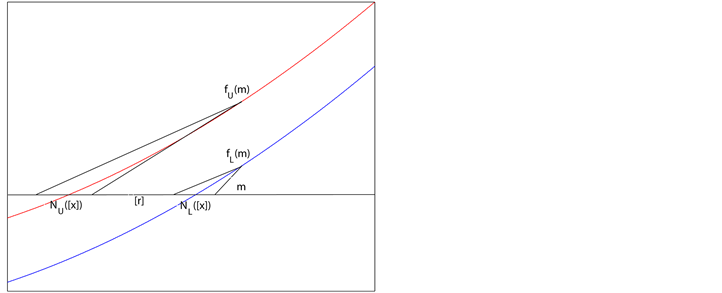Figure 2. Two cases: [r] not existing or existing in lemma 2.2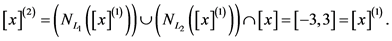3. 新的改进算法

1) 若，则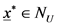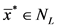2) 若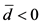，则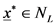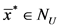3) 若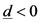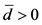，根据Hansen  提出的处理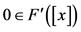的情况有：(8)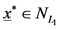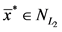4) 若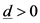，同理有：(9)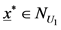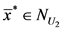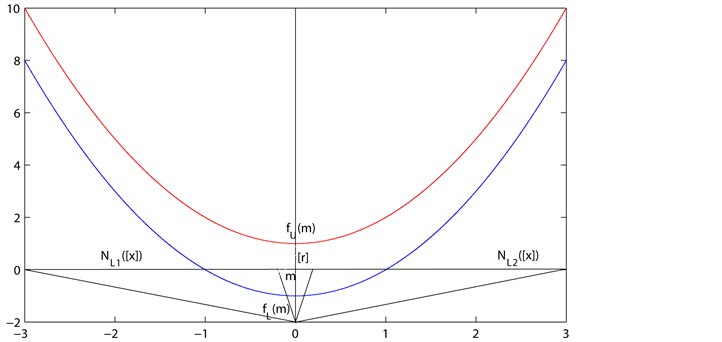Figure 3. Interval equation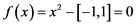,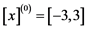4. 数值算例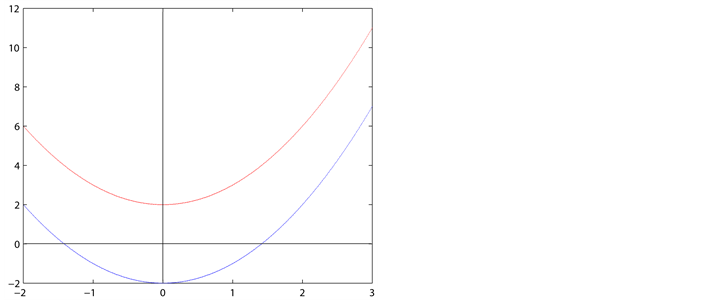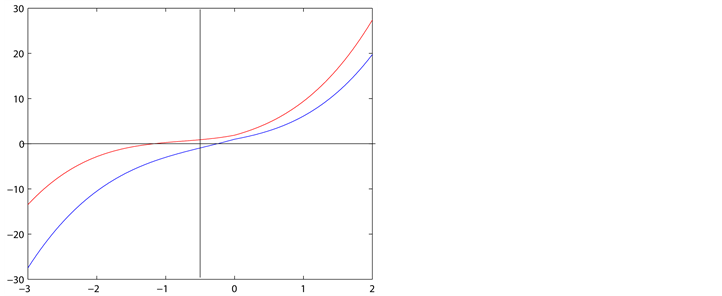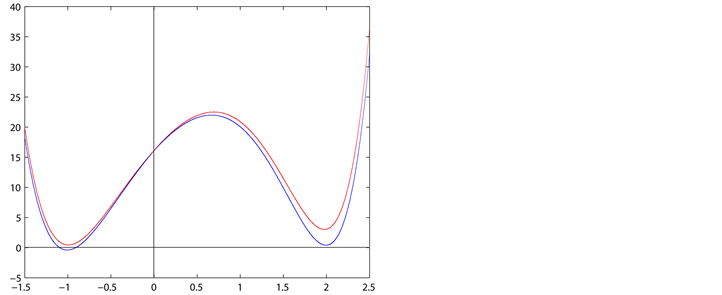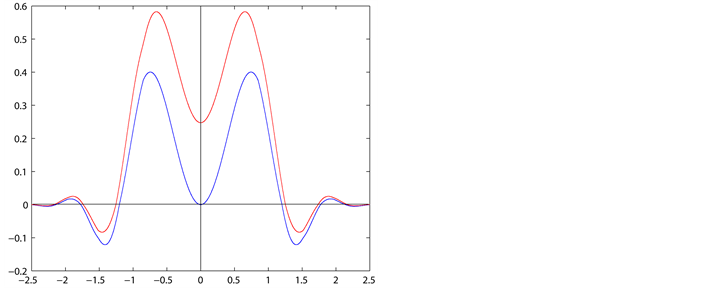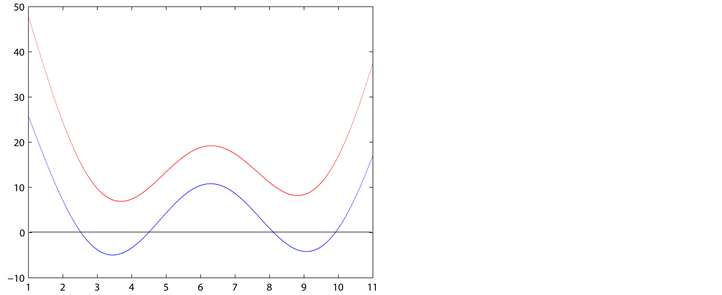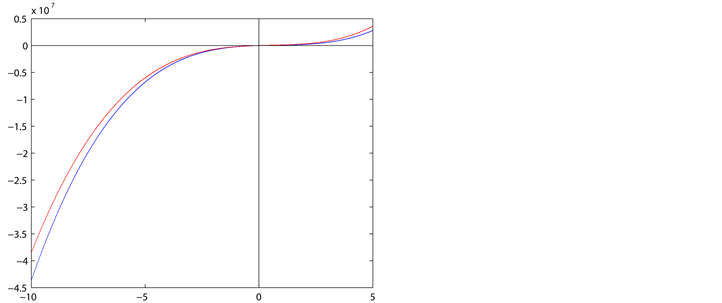Figure 4. The image of test functionTable 3. Numerical results are calculated by algorithm1

Continued

5. 总结

A Class of Improved Algorithm for Solving Interval Nonlinear Equation[J]. 应用数学进展, 2017, 06(05): 716-725. http://dx.doi.org/10.12677/AAM.2017.65086

1. 1. Nikas, I. and Grapsa, T. (2009) Bounding the Zeros of an Interval Equation. Applied Mathematics and Computation, 213, 466-478. https://doi.org/10.1016/j.amc.2009.03.041

2. 2. Balaji, G. and Seader, J. (1995) Application of Interval Newton’s Method to Chemical Engineering Problems. Reliable Computing, 1, 215-223. https://doi.org/10.1007/BF02385253

3. 3. Carpani, O. Hvidegaard, L., Mortensen, M. and Schneider, T. (2000) Robust and Efficient Ray Intersection of Implicit Surfaces. Reliable Computing, 6, 9-21. https://doi.org/10.1023/A:1009921806032

4. 4. The COPRIN Project (2003) Minimal and Maximal Real Roots of Parametric Polynomials Using Interval Analysis. In: Bliek, C., Jermann, C. and Neumaier, A., Eds., Global Optimization and Constraint Satisfaction, First International Workshop Global Constraint Optimization and Constraint Satisfaction, COCOS 2002, Valbonne-Sophia Antipolis, France, October 2-4, 2002, Revised Selected Papers, Lecture Notes in Computer Science, Vol. 2861, Springer.

5. 5. Šiljak, D. and Stipanović, D. (1999) Robust D-Stability via Positivity. Automatica, 35, 1477-1484. https://doi.org/10.1016/S0005-1098(99)00042-4

6. 6. Moore, R. (1966) Interval Analysis. Prentice-Hall, Inc., Englewood Cliffs, New Jersey.

7. 7. Hansen, E. (1992) Global Optimization Using Interval Analysis. Monographs and Textbooks in Pure and Applied Mathematics, Vol. 165, Marcel Dekker, New York.

8. 8. Nikas, I. Sotiropoulos, D. and Grapsa, T. (2006) Extending Interval Newton Method for Nonlinear Parameterized Equations. In: Simos, T., Psihoyios, G. and Tsitouras, C., Eds., ICNAAM-International Conference on Numerical Analysis and Applied Mathematics, Wi-ley-VCH, Hersonisos, Crete, 512-515, ISBN 3-527-40743-X.

9. 9. Hansen, E. (1978) Interval Forms of Newton’s Method. Computing, 20, 153-163. https://doi.org/10.1007/BF02252344